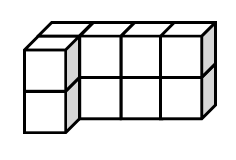### Home > CC3MN > Chapter 9 > Lesson 9.1.3 > Problem9-47

9-47.

Find the surface area and volume of the prism at the right.

Surface area is the total area of the surfaces of an object.

Volume is the space that an object occupies:
To find the volume of a prism, multiply the volume of one layer by the number of layers.

$10$ cubes make up this object.
How many sides act as a surface for the object and count towards the surface area?

Surface Area $= 34$ square units
Volume $= 10$ cubic units